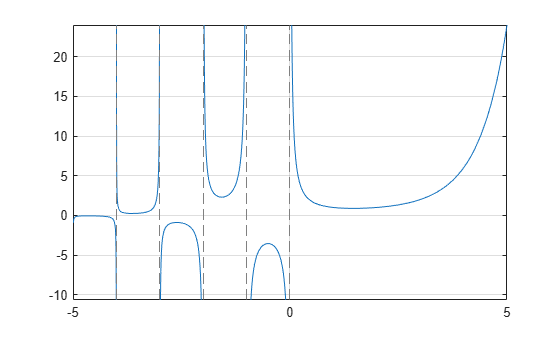# gamma

Gamma function

## Syntax

``gamma(X)``

## Description

example

````gamma(X)` returns the gamma function of a symbolic variable or symbolic expression `X`.```

## Examples

### Gamma Function for Numeric and Symbolic Arguments

Depending on its arguments, `gamma` returns floating-point or exact symbolic results.

Compute the gamma function for these numbers. Because these numbers are not symbolic objects, you get floating-point results.

`A = gamma([-11/3, -7/5, -1/2, 1/3, 1, 4])`
```A = 0.2466 2.6593 -3.5449 2.6789 1.0000 6.0000```

Compute the gamma function for the numbers converted to symbolic objects. For many symbolic (exact) numbers, `gamma` returns unresolved symbolic calls.

`symA = gamma(sym([-11/3, -7/5, -1/2, 1/3, 1, 4]))`
```symA = [ (27*pi*3^(1/2))/(440*gamma(2/3)), gamma(-7/5),... -2*pi^(1/2), (2*pi*3^(1/2))/(3*gamma(2/3)), 1, 6] ```

Use `vpa` to approximate symbolic results with floating-point numbers:

`vpa(symA)`
```ans = [ 0.24658411512650858900694446388517,... 2.6592718728800305399898810505738,... -3.5449077018110320545963349666823,... 2.6789385347077476336556929409747,... 1.0, 6.0] ```

### Plot Gamma Function

Plot the gamma function and add grid lines.

```syms x fplot(gamma(x)) grid on```### Handle Expressions Containing Gamma Function

Many functions, such as `diff`, `limit`, and `simplify`, can handle expressions containing `gamma`.

Differentiate the gamma function, and then substitute the variable t with the value 1:

```syms t u = diff(gamma(t^3 + 1)) u1 = subs(u, t, 1)```
```u = 3*t^2*gamma(t^3 + 1)*psi(t^3 + 1) u1 = 3 - 3*eulergamma```

Approximate the result using `vpa`:

`vpa(u1)`
```ans = 1.2683530052954014181804637297528```

Compute the limit of the following expression that involves the gamma function:

```syms x limit(x/gamma(x), x, inf)```
```ans = 0```

Simplify the following expression:

```syms x simplify(gamma(x)*gamma(1 - x))```
```ans = pi/sin(pi*x)```

## Input Arguments

collapse all

Input, specified as symbolic number, variable, expression, function, or as a vector or matrix of symbolic numbers, variables, expressions, or functions.

collapse all

### Gamma Function

The following integral defines the gamma function:

`$\Gamma \left(z\right)=\underset{0}{\overset{\infty }{\int }}{t}^{z-1}{e}^{-t}dt.$`